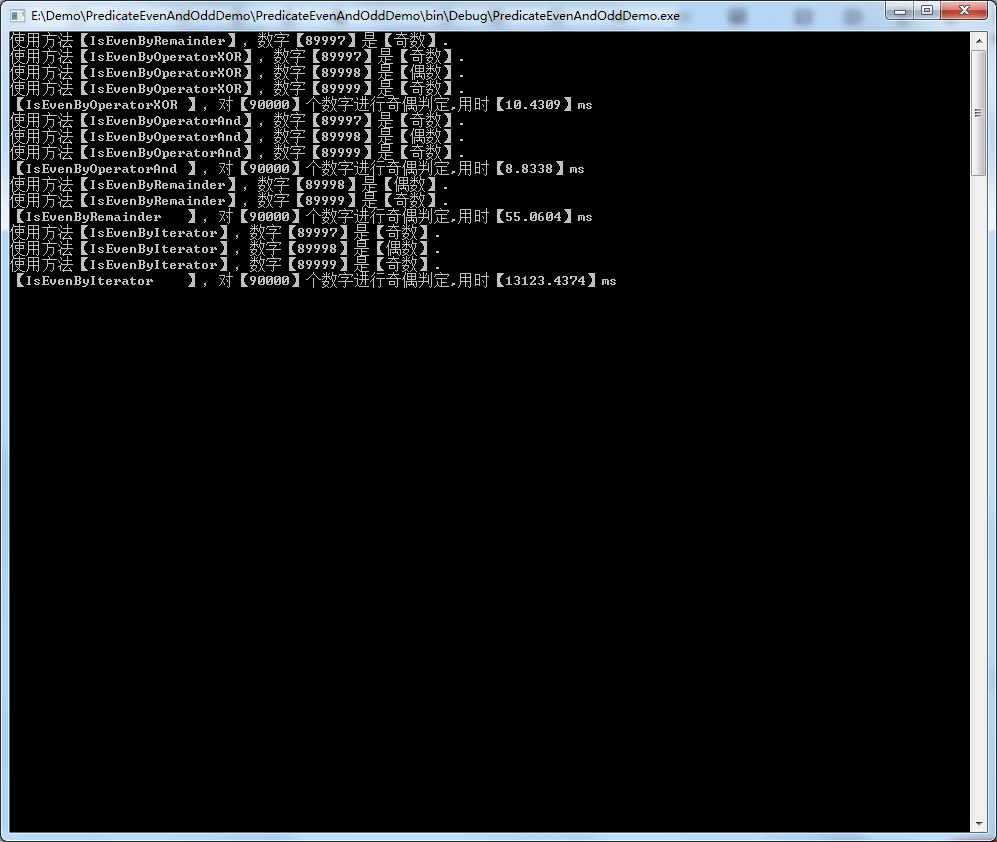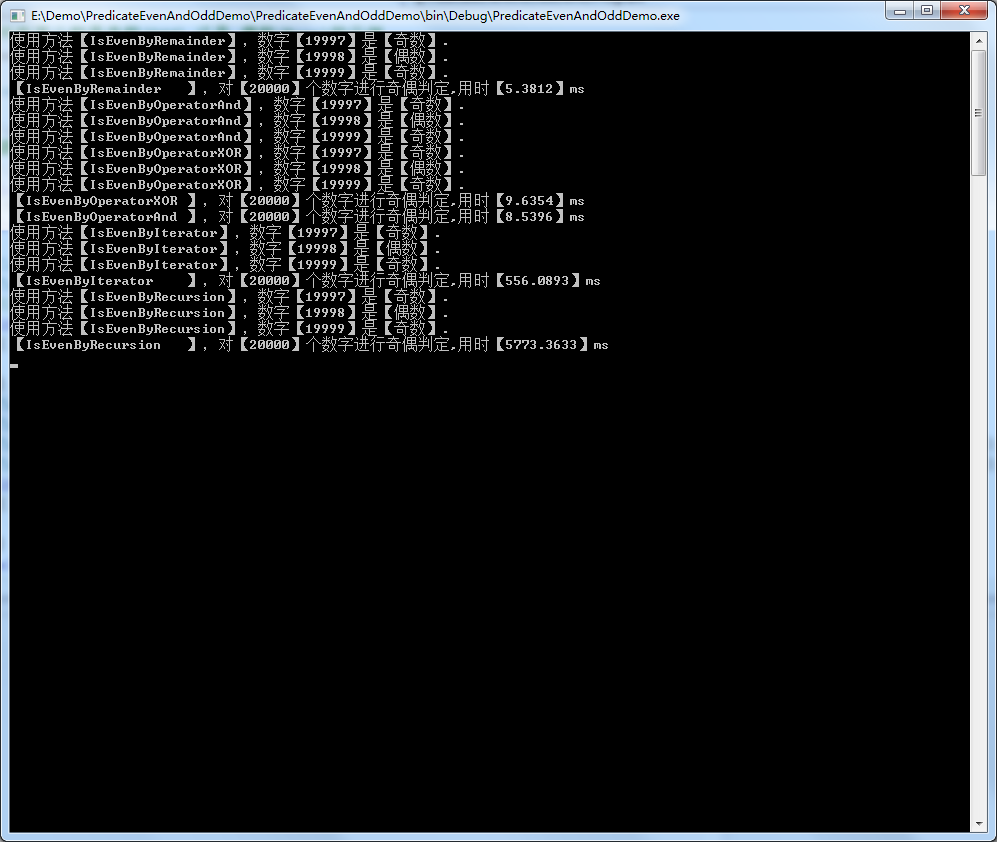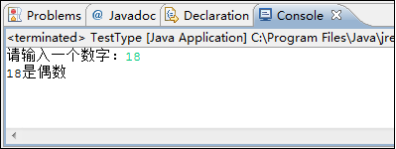• python3.7 输入一个整数判断是否为偶数 print("判断一个数是否为偶数") number=input("请输入一个整数：") time=1 while(time==1): while(not (number.isdigit())): print('这不是一个整数，请重新输入：',end...
python3.7 输入一个整数，判断其是否为偶数
print("判断一个数是否为偶数")
number=input("请输入一个整数：")
time=1
while(time==1):
while(not (number.isdigit())):
print('这不是一个整数，请重新输入：',end=' ')
number=input()
number=int(number)
a=number/2
b=int(a)
if(a-b>0):
print(str(number)+"不是偶数。")
else:
print(str(number)+'是偶数。')
print("重新判断请输入‘1’：",end=" ")
time=input()
if(time.isdigit()):
time=int(time)
if(time==1):
number=input('请输入一个整数：')
print('判断结束！')

不足之处请大家积极指正。
展开全文python
• 方法 #include <bits/stdc++.h> using namespace std; ... else printf("%d是偶数\n",x); x=9; if(x&1) { printf("%d是奇数\n",x); } else printf("%d是偶数\n",x); return 0
方法
#include <bits/stdc++.h>

using namespace std;
int main()
{
int x = 6;
if(x&1)
{
printf("%d是奇数\n",x);
}
else printf("%d是偶数\n",x);
x=9;
if(x&1)
{
printf("%d是奇数\n",x);
}
else printf("%d是偶数\n",x);
return 0;
}


作用：
这个方法很重要，在一些题如果用x%2可能会超时
展开全文• 列举出几种判断某数是否偶数的方式 以及执行10000万次，计算其算法用时。 第一种：取余 即 num%2==0 第二种，库函数 Math.IsOdd(num) 第三种，位运算符& 即(num&1)==0 第四种，异或运算符^ 即 (num^1) =...
一个整数能被2整除，这个数就是偶数，
如果不能被2整除【余数为1】，这个数就是奇数
列举出几种判断某数是否偶数的方式 以及执行几万次，计算其算法用时。
第一种：取余 即 num%2==0
第二种，位运算符&  即 (num&1)==0
第三种，异或运算符^ 即 (num^1) == num+1
异或^也是将数字转换成二进制运算，只要两者不同才为1，否则为0（运算规则：0^0=0; 0^1=1; 1^0=1; 1^1=0;）,011^001=010等价于十进制的2，所以为奇数，偶数运算后等于本身加1.
第四种，循环（迭代器）
每次减去2，直到为1 或 0 为止
第五种，递归
return IsEven(num - 2)，直到为1 或 0 为止
新建控制台应用程序PredicateEvenAndOddDemo，
测试源程序如下：
using System;
using System.Collections.Generic;
using System.Diagnostics;
using System.Linq;
using System.Text;

namespace PredicateEvenAndOddDemo
{
/// <summary>
/// 一个判断某个数是奇数(Odd) 还是 偶数(Even)的示例
/// </summary>
class Program
{
/// <summary>
/// 最大测试数，可根据实际情况进行修改
/// </summary>
static int maxNumber = 90000;
static void Main(string[] args)
{
Console.SetWindowSize(120, 50);
//考虑到递归数太大会导致堆栈溢出
if (maxNumber <= 20000)
{
}
if (maxNumber <= 20000)
{
}
else
{
}
}

/// <summary>
/// 算法用时
/// </summary>
/// <param name="PredicateMethod">判定是否是偶数的方法</param>
/// <param name="methodName"></param>
static void AlgorithmTimespan(Func<int, bool> PredicateMethod, string methodName)
{
Stopwatch stopwatch = Stopwatch.StartNew();
for (int number = 0; number < maxNumber; number++)
{
bool isEven = PredicateMethod(number);
if (number >= maxNumber - 3)
{
Console.WriteLine($"使用方法【{methodName}】，数字【{number}】是【{(isEven ? "偶数" : "奇数")}】."); } } Console.WriteLine($"【{methodName.PadRight(20)}】，对【{maxNumber}】个数字进行奇偶判定,用时【{stopwatch.Elapsed.TotalMilliseconds}】ms");
}

/// <summary>
/// 【判断是否偶数】方法一：除以2后判断余数是否为0
/// </summary>
/// <param name="number"></param>
/// <returns></returns>
static bool IsEvenByRemainder(int number)
{
number = Math.Abs(number);
return number % 2 == 0;
}

/// <summary>
/// 【判断是否偶数】方法二：使用与操作符【And】
/// </summary>
/// <param name="number"></param>
/// <returns></returns>
static bool IsEvenByOperatorAnd(int number)
{
number = Math.Abs(number);
return (number & 1) == 0;
}

/// <summary>
/// 【判断是否偶数】方法三：使用异或操作符【XOR】
/// 偶数转化成二进制，最低位一定为0
/// </summary>
/// <param name="number"></param>
/// <returns></returns>
static bool IsEvenByOperatorXOR(int number)
{
number = Math.Abs(number);
return (number ^ 1) == number + 1;
}

/// <summary>
/// 【判断是否偶数】方法四：使用迭代器【循环】,每次减少2，直到小于2为止
/// </summary>
/// <param name="number"></param>
/// <returns></returns>
static bool IsEvenByIterator(int number)
{
number = Math.Abs(number);
while (number > 1)
{
number = number - 2;
}
return number == 0;
}

/// <summary>
/// 【判断是否偶数】方法五：使用递归，每次减少2，直到小于2为止
/// </summary>
/// <param name="number"></param>
/// <returns></returns>
static bool IsEvenByRecursion(int number)
{
number = Math.Abs(number);
if (number == 0)
{
return true;
}
else if (number == 1)
{
return false;
}
return IsEvenByRecursion(number - 2);
}
}
}

运行截图如下：
一般来说：
算法用时：递归 > 循环 > 四则运算 > 位运算
其中 四则运算 与 位运算 并没有很大的差别。反而循环次数的多少会严重影响算法优劣，其中递归的堆栈操作占用
【90000】个运行结果【20000】个运行结果展开全文算法
• 需求说明：正确使用条件运算符判断一个数字是否为偶数偶数的定义是可以被2整除的数，即这个数“%2”，结果0就是偶数，程序执行效果参考下图。package 作业;import java.util.Scanner;public class 判断是否为...
需求说明：正确使用条件运算符判断一个数字是否为偶数，偶数的定义是可以被2整除的数，即这个数“%2”，结果为0就是偶数，程序执行效果参考下图。package 作业;

import java.util.Scanner;

public class 判断是否为偶数 {

public static void main(String[] args) {
// TODO Auto-generated method stub
System.out.println("请输入一个数字：");
Scanner scanner= new Scanner(System.in);
int num = scanner.nextInt();
String result =(num%2==0)? "是偶数":"不是偶数";
System.out.println(num+result);
}

}
展开全文• 面试题：判断一个整数是否为偶数，要求使用至少3种方法 刚来上海没几天，正在找工作，找工作的过程中遇到一家公司的面试题： 判断一个整数是否为偶数，要求使用至少3种方法 时间仓促，当时只想到2种...
• 1、对一个数位与1是用来取位，n&1就可以将数字n最低位取出，如果n最低位是0（这里指n的二进制表示，所有的数在内存均是以二进制形式表示），那么表示n为偶数，因为最低位0，表示可以整除2，这是二进制常识（如果不...
• 1. 如何快速的判断一个数是否偶数 分析： 我们的第一想法一定是这个数取余2 0 则是偶数 1 则是奇数 代码如下： import java.util.Scanner; /** * Class day01 ... * * @author LiJun * Created on ...
• import java.util.Scanner; public class Test0{ public static void main(String[] args) { Scanner sca = new Scanner(System.in);...System.out.println("输入一个整数:"); int intValue =
• #Python3 实例--以下实例用于判断一个数字是否为奇数或偶数： print("Python3 实例--以下实例用于判断一个数字是否为奇数或偶数：") def joshu(x): if x % 2 == 0: print("{}是偶数".format(x)) else: print("{...python
• 1、传统的利用取余进行判断 if (n % 2 == 1) { //n奇数 } if (n % 2 == 0) { //n奇数 } 2、利用位运算&进行判断 if((n & 1) == 1){ // n 是个奇数。 } if((n & 1) == 0){ // n 是个偶数。 ...位运算 异或运算
• C实现一个函数，计算N个整数中所有偶数的和，并实现一个判断奇偶性的函数 函数接口定义： int even( int n ); int EvenSum ( int List[], int N ); 其中函数even将根据用户传入的参数n的奇偶性返回相应值：当n为偶数...c语言
• 用三元运算符实现奇偶数判断，程序要求用户输入一个整数，然后程序判断是奇数还是偶数，并输出到控制台中。代码如下： import java.util.Scanner; /** * 用三元运算符判断是奇书还是偶数 */ public class ...Java经典编程案例
• 若 x 是偶数，则函数值 1(真)，否则 0(假)。 裁判程序 #include <stdio.h> int IsEven(int x); int main() { int a; scanf("%d", &a); if (IsEven(a)) { puts("Yes"); } elsc语言
• ## vb2010 偶数判断函数

千次阅读 2012-05-06 21:09:22
Public Function 偶数判断(ByVal 需要判断的数字 As Long) As Boolean '判断是不是偶数，就可以知道是不是奇数了 Dim 返回结果 As Boolean = True If 需要判断的数字 Mod 2 = 0 Then 返回结果 = True Else 返回...function
• #include<stdio.h> int main() { int a,b; scanf("%d",&a); printf("%d",a>100||a<0); }算法 c++ c语言
• 利用if-else语句判断整数的正负和奇偶 import java.util.Scanner; public class java_07 { public static void main(String[] args) { Scanner scan = new Scanner(System.in); System.out.println("请输入一...算法 java
• ## SQL语句判断奇偶数

千次阅读 2021-03-19 15:01:31
请你查找employees表所有emp_no奇数，且last_name不Mary的员工信息，并按照hire_date逆序排列，以上例子查询结果如下: 方法一：使用mod() mod(a,b) 在sql中的意思是 a / b 的余数 mod(id, 2)=1 是指id是奇数。 ...sql
• x >> y 表示x/（2的y次方） ...10 |3 可以看作 1010 |0011 每位做或运算 结果1011=11 这里说明 位运算是 优于 求余运算的 可是在测试的时候可能会发现二者运算时间差的不是很大，只有很小的...
• Python：判断奇数偶数 n=eval(input('输入数字：')) if n%2==1: print('odd number') elif n%2==0: print('even number') else: print('请输入整数')python
• ## python判断奇数偶数

万次阅读 2019-08-21 15:08:36
print('{0}是个偶数'.format(num)) else: print('%d是个奇数'%num) 加上错误异常处理版本 如果输入有错，跳过判断重新输入 如果输入正确，判断一次之后跳出循环 while True: try: num = int(i...python
• #include<stdio.h> int main() { int a; scanf("%d",&a); if (a%2==0) { printf("even"); } else { printf("odd"); } return 0; }c语言 算法
• 输入一个整数判断是奇数还是偶数 输入 输入1个正整数 输出 如果是偶数，输出even否则输出odd 样例输入 Copy 2 样例输出 Copy even 下面是代码 //创作者：小小阁楼 #include<stdio.h> #include<math.h&...c++
• import java.util.Scanner; public class ThreeUnary { ... "偶数":"奇数"; //如果num的余数0时，num为偶数，否则奇数。  System.out.println(num1); //求出num1   } }
• //利用三元运算符对此输入数字进行判断是奇数还是偶数 public class jiO { public static void main(String[] args) { System.out.println("请输入一个整数："); long l = new Scanner(System.in).nextLong(); ...java
• 1、语法： if commands; then commands; [else if commands; then commands;... 计算其后的命令即 commands的退出状态是成功还是失败，并据此判断下一步该执行哪些commands &amp;gt;if ...shell
•  System.out.println("请输入任意一个整数：");  long n;  n = scan.nextLong();  String rst = n%2==0?"是偶数!":"不是偶数!";  System.out.print(n+rst+" ");  System.err.println("2011.11.8 ...string import class java
• 为了简化问题和突出重点，这里我们假设脚本的输入参数一定合法的整数类型，因而在脚本内部将不再进行参数的合法性判断。 [root@xieqichao ~]# cat > test4.sh #!/bin/sh #1. 这里的重点主要是sed命令中正则...
• public class Test{  public static void main(String[] args) {  Scanner scanner = new Scanner(System.in);... System.out.println("请输入一个整数：");  long num =scanner.nextLong();
• 给定一个整数判断该数是奇数还是偶数。 输入 输入仅一行，一个大于零的正整数n。 输出 输出仅一行，如果n是奇数，输出odd；如果n是偶数，输出even。 样例输入 5 样例输出 odd #include<iostream> #include&...c++ c语言
• 是回文数的判断条件：加入一个变量位数，如果这个数是奇数，位数1时，即最中间那一位数，此时退出即可，同理，偶数，位数0时，退出。 问题： 如何判断位数 如何逐位取值 优点： 思路简单 解决： 判断位数下面...python 回文数...